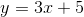# High School Math : How to find the slope of parallel lines

## Example Questions

### Example Question #1 : How To Find The Slope Of Parallel Lines

Which of the following lines would be parallel to the line described by the equation?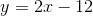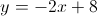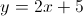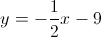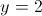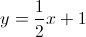Explanation:

The way to determine parallel lines is to look at the slope. That means when you look at the equation in slope-intercept form,, you're looking at theIn the given problem, the slope is. Parallel lines will have identical slopes; thus, any line that is parallel to the line described by the equation would ALSO have a slope of. Only one answer choice satisfies that requirement:.

### Example Question #1 : How To Find The Slope Of Parallel Lines

Which of the following lines would be parallel to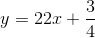?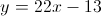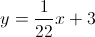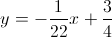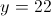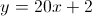Explanation:

Two lines are parallel if they have the same slope. When looking at the standard line equation, the important thing is that the's are the same. In this case, the given equation has a slope of. Only one answer choice also has a slope of.

### Example Question #3 : How To Find The Slope Of Parallel Lines

What is the slope of the line that runs through points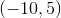and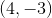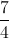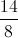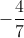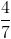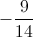Explanation:

Use the slope formula (difference between's over difference between's) to find that the slope is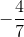.

### Example Question #1 : How To Find The Slope Of Parallel Lines

A line that is parallel to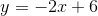will have what slope?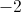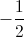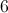Explanation:

Two lines that are parallel have the same slope. The line given above is in slope-intercept form,, whererepresents the slope. Thus, the slope is. Therefore, any line that is parallel to this line will also have a slope of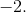### Example Question #5 : How To Find The Slope Of Parallel Lines

Which of the following equations are parallel to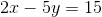?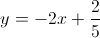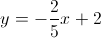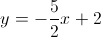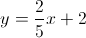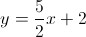Explanation:

For one equation to be parallel to another, the only requirement is that they must have the same slope. In order to figure out which answer choice is parallel to the given equation, you must first find the slope of the equation: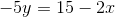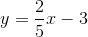From the simplified equation, you can see that the slope is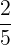.

The answer choice that has the same slope is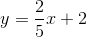.

### Example Question #2 : How To Find The Slope Of Parallel Lines

Which of the following is parallel to a line described by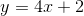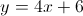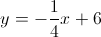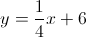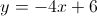Explanation:

The definition of parallel lines tells us that if two lines are parallel, they have the same slope. Since these lines are described in the slope-intercept form, we know that the slope of these lines are given by the coefficient of thevariable.

The slope in the given equation is 4, so a parallel line would also have a slope of 4. The only answer with this slope is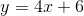### Example Question #7 : How To Find The Slope Of Parallel Lines

Which of the following is parallel to the line described by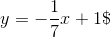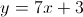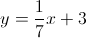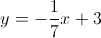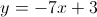Explanation:

The definition of parallel lines tells us that if two lines are parallel, they have the same slope. Since these lines are described in the slope-intercept form, we know that the slope of these lines are given by the coefficient of thevariable.

The slope in the given equation is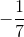, so a parallel line would also have a slope of. The only answer with this slope is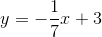### Example Question #3 : How To Find The Slope Of Parallel Lines

Which of the following is parallel to the line described by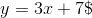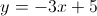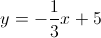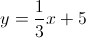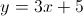The definition of parallel lines tells us that if two lines are parallel, they have the same slope. Since these lines are described in the slope-intercept form, we know that the slope of these lines are given by the coefficient of thevariable.